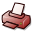# Variational Hamiltonian Approach to the Quark Sector of QCD in Coulomb Gauge

## DSpace Repository

 Dateien: 4.32 MB PDF Description: Dissertation

 URI: Dokumentart: Dissertation Date: 2018-07-09 Language: English Faculty: 7 Mathematisch-Naturwissenschaftliche Fakultät Department: Physik Advisor: Reinhardt, Hugo (Prof. Dr. ) Day of Oral Examination: 2018-06-25 DDC Classifikation: 530 - Physics Keywords: Quantenchromodynamik , Coulomb-Eichung , Graphen , Symmetriebrechung Other Keywords:QCDCoulomb GaugeChiral Symmetry BreakingGraphene License: Order a printed copy:Print-on-Demand Show full item record

### Abstract:

Despite being considered as the correct theory for describing the interaction between gluons and quarks, Quantum Chromodynamics (``QCD'') still has elusive features which should be investigated. Although the theory can be dealt with at high energy by means of perturbation theory, at low energies one needs non-perturbative methods for investigating phenomena such as confinement and chiral symmetry breaking. One possible method which has been used in this thesis is the variational Hamiltonian approach. In this method, after considering an ansatz for the vacuum wave functional and calculating the vacuum energy by taking the vacuum expectation value of the Hamiltonian, the variational method can be applied which leads to the gap equation. A non-trivial solution of this equation can be considered as a sign of chiral symmetry breaking. In this thesis, after an overview of Yang-Mills theory and QCD, I introduce the Hamiltonian approach to the QCD in Coulomb gauge. The ansatz for the vacuum wave functional is introduced and the vacuum energy for the quark sector of QCD is calculated. Next, the gap equation for the quarks both in the absence and presence of the coupling to transverse gluons is derived. These gap equations are then solved numerically and the quark condensate as an order parameter for the spontaneous breaking of chiral symmetry is calculated. Furthermore, the calculations are extended to finite temperatures by compactification of a spatial dimension. The compactified gap equation in the absence of the transverse gluons is solved and the quark condensate at finite temperatures is calculated. Finally, the great flexibility of the variational Hamiltonian approach is demonstrated by applying it to an interesting question in solid state physics, namely the electronic correlations in graphene.